Courses

# Test: Basic Algebra

## 10 Questions MCQ Test Quantitative Aptitude for GMAT | Test: Basic Algebra

Description
This mock test of Test: Basic Algebra for GMAT helps you for every GMAT entrance exam. This contains 10 Multiple Choice Questions for GMAT Test: Basic Algebra (mcq) to study with solutions a complete question bank. The solved questions answers in this Test: Basic Algebra quiz give you a good mix of easy questions and tough questions. GMAT students definitely take this Test: Basic Algebra exercise for a better result in the exam. You can find other Test: Basic Algebra extra questions, long questions & short questions for GMAT on EduRev as well by searching above.
QUESTION: 1

### A poultry farm has only chickens and pigs. When the manager of the poultry counted the heads of the stock in the farm, the number totaled up to 200. However, when the number of legs was counted, the number totaled up to 540. How many more chickens were there in the farm? Note: In the farm, each pig had 4 legs and each chicken had 2 legs.

Solution:

Step 1 : Assign Variables and Frame Equations
Let the number of chickens in the farm be 'x'.
Let the number of pigs in the farm be 'y'.

Each pig or each chicken has one head.
Therefore, number of heads will be the same as the sum of the chickens and pigs in the farm.
The count of the heads in the farm is 200. So, the sum of the number of chickens and pigs is 200.
So, x + y = 200 .... (1)

Each chicken has 2 legs and each pig has 4 legs
'x' chickens will therefore, have 2x legs and 'y' pigs will have 4y legs.
The count of the legs in the farm is 540. So, the sum of the number of legs of chickens and the number of legs of pigs is 540.
Therefore, 2x + 4y = 540 .... (2)

Step 2 of solving this GMAT Linear Equations Question: Solve the system of linear equations
Multiply equation (1) by 2. We get 2x + 2y = 400 .... (3)
Subtract equation (3) from equation (2).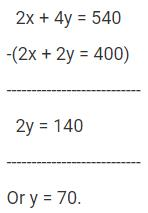Substitute 'y' as 70 in equation (1).
70 + y = 200. So, x = 130

'x' is the number of chickens in the farm. 'y' is the number of pigs in the farm. So, the farm has 130 chickens and 70 pigs.

Note:The question is "How many more chickens were there in the farm?"
The farm has 130 chickens and 70 pigs. So, the farm has (130 - 70) = 60 more chickens

QUESTION: 2

### Three years back, a father was 24 years older than his son. At present the father is 5 times as old as the son. How old will the son be three years from now?

Solution:

Let the age of the son 3 years back be 'x' years.
Information 1: Three years back, a father was 24 years older than his son.
Therefore, the age of the father 3 years back was (x + 24).

If the age of the son 3 years back was 'x' years, the present age of the son is x + 3.
Present age of father = x + 24 + 3

Information 2: The father is at present 5 times as old as the son.
i.e., (x + 24 + 3) = 5(x + 3)
Or x + 27 = 5x + 15
Or 4x = 12 or x = 3.

Step 2 of solving this GMAT Algebra Word Problem: From unknown to the answer
x was the age of the son 3 years back.
Therefore, the son was 3 years old 3 years back.

The question is "How old will the son be three years from now?"
If the son was 3 years old, 3 years back, the son is 6 years old now.
Hence, he will be 9 years old three years from now.

Choice D is the correct answer.

QUESTION: 3

### For what values of 'k' will the pair of equations 3x + 4y = 12 and kx + 12y = 30 NOT have a unique solution?

Solution:

A system of linear equations ax + by + c = 0 and dx + ey + g = 0 will have a unique solution if the two lines represented by the equations ax + by + c = 0 and dx + ey + g = 0 intersect at a point.
i.e., if the two lines are neither parallel nor coincident.
Essentially, the slopes of the two lines should be different.

What does that translate into?
ax + by + c = 0 and dx + ey + g = 0 will intersect at one point if their slopes are different.
Express both the equations in the standardized y = mx + c format, where 'm' is the slope of the line and 'c' is the y-intercept.

ax + by + c = 0 can be written as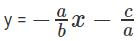And dx + ey + g = 0 can be written as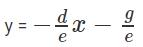Slope of the first line is -a/b and that of the second line is -d/e

For a unique solution, the slopes of the lines should be different.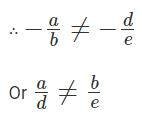Condition for the equations to NOT have a unique solution
The slopes should be equal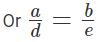Apply the condition in the given equations to find k
In the question given above, a = 3, b = 4, d = k and e = 12.
Therefore,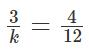Or 'k' should be equal to 9 for the system of linear equations to NOT have a unique solution.

The question is "What is the value of k?
When k = 9, the system of equations will represent a pair of parallel lines (their y-intercepts are different). So, there will be NO solution to this system of linear equations in two variables.
Choice A is the correct answer.

QUESTION: 4

The basic one-way air fare for a child aged between 3 and 10 years costs half the regular fare for an adult plus a reservation charge that is the same on the child's ticket as on the adult's ticket. One reserved ticket for an adult costs \$216 and the cost of a reserved ticket for an adult and a child (aged between 3 and 10) costs \$327. What is the basic fare for the journey for an adult?

Solution:

Step 1 : Assign variables and frame equations
Let the basic fare for the child be \$X.

Information 1: Basic one-way air fare of a child costs half the regular fare for an adult.
Therefore, the basic fare for an adult = 2(basic one-way airfare for a child) = \$2X.

Information 2: Reservation charge is the same on the child's ticket as on the adult's ticket.
Let the reservation charge per ticket be \$Y
A child's ticket will cost (Basic fare + Reservation charges) = X + Y
Hence, an adult ticket will cost (Basic fare + Reservation charges) = 2X + Y.
Information 3: One reserved ticket for an adult costs \$216. So, 2X + Y = \$216 .... (1)
Information 4: The cost of a reserved ticket for an adult and a child (aged between 3 and 10) is \$327.
So, the ticket for an adult and a child will cost (2X + Y) + (X + Y) = 3X + 2Y = \$327 .... (2)

Step 2 of solving this GMAT Algebra Question: Solve the simultaneous equations and determine basic fare for an adult.
Multiply equation (1) by 2: 4X + 2Y = 432 .... (3)
Subtract equation (2) from equation (3):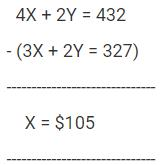The question is "What is the basic fare for an adult?"
The basic fare of an adult ticket = 2X = 2*105 = \$210

Choice C is the correct answer.

QUESTION: 5

This data sufficiency problem consists of a question and two statements, labeled (1) and (2), in which certain data are given. You have to decide whether the data given in the statements are sufficient for answering the question. Using the data given in the statements, plus your knowledge of mathematics and everyday facts (such as the number of days in a leap year or the meaning of the word counterclockwise), you must indicate whether -

Q.

1. (y - 3)(x - 4) = 0
2. (x - 4) = 0

Numbers

All numbers used are real numbers.

Solution:

Step 1

Understand the Question Stem
What kind of an answer will this GMAT DS question fetch?
The question is an "Is" question. Answer to an "is" questions is either YES or NO.

When is the data sufficient?
The data is sufficient if we are able to get a DEFINITE YES or a DEFINITE NO from the information given in the statements.

Do we have any additional information about 'y' from the question stem?
There is no additional data available about 'y' in the question stem.

Step 2 of solving this GMAT system of equations question:
Evaluate Statement (1) ALONE: (y - 3)(x - 4) = 0
If product of the two terms (y - 3) and (x - 4) is 0, then at least one of the two terms equals 0.
(y - 3) = 0 or (x - 4) = 0 or both (y - 3) and (x - 4) equal 0.
i.e., either y = 3 or x = 4 or that both y = 3 and x = 4.
If x = 4, y could take any value. The value of 'y' could be 3 or it could be some other value and the product will still be a 0.
Example: x = 4 and y = 5. The equation holds good. y ≠ 3.
Counter example: x = 4 and y = 3. The equation holds good. y = 3
We CANNOT determine whether 'y' is 3 from this statement.

Statement 1 ALONE is NOT sufficient.
Eliminate choices A and D. Choices narrow down to B, C or E.

Step 3 of solving this GMAT algebra DS question:
Evaluate Statement (2) ALONE: (x - 4) = 0
The statement provides no information about y.

Statement 2 ALONE is NOT sufficient.
Eliminate choice B. Choices narrow down to C or E.

Step 4 of solving this GMAT DS question:
Evaluate Statements (1) & (2) Together: (y - 3)(x - 4) = 0 & (x - 4) = 0
When x = 4, (y - 3)(x - 4) will be 0 irrespective of the value that y takes.
Can 'y' be 3? Yes 'y' can be 3.
Is y = 3? Not necessary.It can take values other than 3 and the data in the two statements will still hold good.

Eliminate choice C.

Statements TOGETHER are NOT sufficient. Choice E is the answer.

QUESTION: 6

A children's gift store sells gift certificates in denominations of \$3 and \$5. The store sold 'm' \$3 certificates and 'n' \$5 certificates worth \$93 on a Saturday afternoon. If 'm' and 'n' are natural numbers, how many different values can 'm' take?

Solution:

Step 1: Understand Key Data

Total value of all certificates sold = \$93.
Certificates sold were in denominations of \$3 and \$5.
Both 'm' and 'n' are natural numbers.
Approach to solve this GMAT Algebra Word Problem
The value of all certificates sold, 93 is divisible by 3.
So, a maximum of 31 \$3 certificates and no \$5 certificates could have been sold.
However, the question states that both 'm' and 'n' are natural numbers.
Hence, at least 1 \$5 certificate should have been sold.

Let us reduce the number of \$3 certificates from theoretical maximum count of 31 by say 'x' and correspondingly increase \$5 certificates by 'y'.
Evidently, 3x = 5y because the value of \$3 certificates reduced should be the same as the value of \$5 certificates increased.

It means that x has to be a multiple of 5 and y has to be a multiple of 3.
Or \$3 certificates reduce in steps of 5 certificates.

Step 2 of solving this GMAT Algebra Question: List down possible values for 'm' and 'n'
The following combinations are possible.

m = 26, n = 3
m = 21, n = 6
m = 16, n = 9
m = 11, n = 12
m = 6, n = 15
m = 1, n = 18
The question is "How many different values can 'm' take?"
Hence, m can take 6 values.

Choice C is the correct answer.

QUESTION: 7

What is the highest integral value of 'k' for which the quadratic equation x2 - 6x + k = 0 will have two real and distinct roots?

Solution:

Step 1:

Nature of Roots of Quadratic Equation Theory

D is called the Discriminant of a quadratic equation.
D = b2 - 4ac for quadratic equations of the form ax2 + bx + c = 0.
If D > 0, roots of such quadratic equations are Real and Distinct
If D = 0, roots of such quadratic equations are Real and Equal
If D < 0, roots of such quadratic equations are Imaginary. i.e., such quadratic equations will NOT have real roots.
In this GMAT sample question in algebra, we have to determine the largest integer value that ‘k’ can take such that the discriminant of the quadratic equation is positive.

Step 2:

Compute maximum value of k that will make the discriminant of the quadratic equation positive
The given quadratic equation is x2 - 6x + k = 0.
In this equation, a = 1, b = -6 and c = k
The value of the discriminant, D = 62 - 4 * 1 * k
We have compute values of 'k' for which D > 0.
i.e., 36 – 4k > 0
Or 36 > 4k or k < 9.

The question is "What is the highest integer value that k can take?"
If k < 9, the highest integer value that k can take is 8.

Choice D is the correct answer.

QUESTION: 8

If one of the roots of the quadratic equation x2 + mx + 24 = 0 is 1.5, then what is the value of m?

Solution:

If 1.5 is a root of the quadratic equation, substituting x = 1.5 in the equation will satisfy the equation.

The given quadratic equation is x2 + mx + 24 = 0
Substitute x = 1.5 in the above equation because 1.5 is a root of the equation.
(1.5)2 + 1.5m + 24 = 0
2.25 + 1.5m + 24 = 0

1.5m = -26.25 Or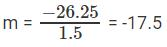QUESTION: 9

For what value of 'm' will the quadratic equation x2 - mx + 4 = 0 have real and equal roots?

Solution:

Step 1:

Nature of Roots of Quadratic Equations Theory

D is the Discriminant in a quadratic equation.
D = b2 - 4ac for quadratic equations of the form ax2 + bx + c = 0.
If D > 0, roots are Real and Unique (Distinct and real roots).
If D = 0, roots are Real and Equal.
If D < 0, roots are Imaginary. The roots of such quadratic equations are NOT real.
The quadratic equation given in this question has real and equal roots. Therefore, its discriminant D = 0.

Step 2:
Compute discriminant for the equation in terms of ‘m’ and find the value of ‘m’.
In the given equation x2 - mx + 4 = 0, a = 1, b = -m and c = 4.
Therefore, the discriminant b2 - 4ac = m2 - 4(4)(1) = m2 - 16.
The roots of the given equation are real and equal.
Therefore, m2 - 16 = 0 or m2 = 16 or m = +4 or m = -4.

QUESTION: 10

y = x2 + bx + 256 cuts the x axis at (h, 0) and (k, 0). If h and k are integers, what is the least value of b?

Solution:

Step 1:

Understand the equation and the points (h, 0) and (k, 0)

The given equation is a quadratic equation. A quadratic equation when plotted on a graph sheet (x - y plane) will result in a parabola.
The roots of the quadratic equation are computed by equating the quadratic expression to 0. i.e., the roots are the values that 'x' take when y = 0
So, the roots of the quadratic equation are the points where the parabola cuts the x-axis.
The question mentions that the curve described by the equation cuts the x-axis at (h, 0) and (k, 0). So, h and k are the roots of the quadratic equation.
For quadratic equations of the form ax2 + bx + c = 0, the sum of the roots = -b/a

The sum of the roots of this equation is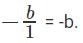Note : Higher the value of 'b', i.e., higher the sum of the roots of this quadratic equation, lower the value of b.

For quadratic equations of the form ax2 + bx + c = 0, the product of roots = c/a

Therefore, the product of the roots of this equation = 256/1 = 256

i.e., h * k = 256 h and k are both integers.
So, h and k are both integral factors of 256.

Step 2 :

List possible values of h and k and find the least value of ‘b’
This is the step in which number properties kicks in. 256 can be expressed as product of two numbers in the following ways:
1 * 256
2 * 128
4 * 64
8 * 32
16 * 16

The sum of the roots is maximum when the roots are 1 and 256 and the maximum sum is 1 + 256 = 257.
∴ The least value possible for b is -257.

Choice D is the correct answer.